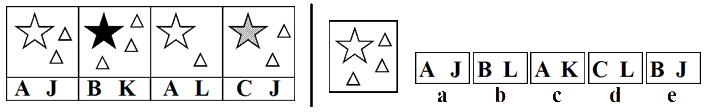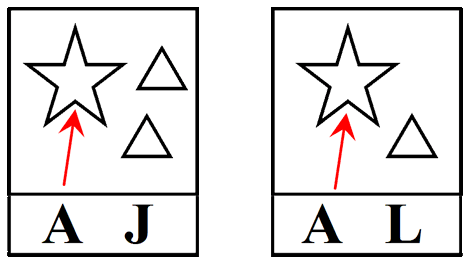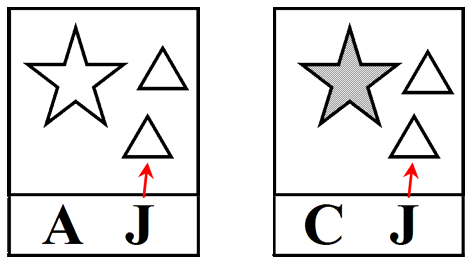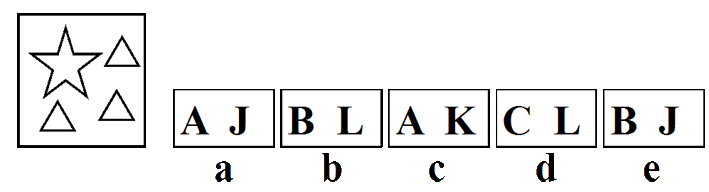Type 4 - 'Code Breaker'

With this type of question you are given four boxes to the left of a vertical line containing symbols or shapes. Associated with each of the boxes are two letters. To the right of the vertical line is another box containing symbols and a set of five pairs of letters, labelled a to e.

The letters associated with the boxes are a code that determines what symbols or shapes are contained within the box.

You are required to work out the code - ie. what symbol/shape does each letter represent? After identifying the code, you need to decide which letters would represent the shapes and symbols in the box to the right of the vertical line.

Remember that the figures and diagrams consist of lines, shapes and shading/patterns. The shapes can shift in various ways. (rotate, reflect and transpose). The shapes can change in many ways. (increase/decrease, add and copy). They can consist of a movement, size or number sequence.Begin by looking at the first letter of all the pairs and see if any are the same. In this case the letter 'A' occurs in two of the boxes. Now focus on these two boxes and look for similarities in the shapes and symbols.Both boxes contain one white star each and different numbers of white triangles. The similarity is the one white star - so we can infer that the letter 'A' means 'one white star' and the first letter relates to the type of star.

Looking at the second letters relating to these two boxes we can see that they are different and that the number of white triangles is different. The second letter could relate to the number of triangles.

Now look at the second letter of all the pairs and see if any are the same. In this case the letter 'J' occurs in two of the boxes. Now focus on these two boxes and look for similarities in the shapes and symbols.Both boxes contain different stars and two white triangles each. The similarity is the two white triangles - so we can infer that the letter 'J' means 'two white triangles' and the second letter relates to the number of white triangles.

So now we know that the first letter relates to the type of star and the second letter relates to the number of white triangles.

First letters - A = white star, B = black star and C = shaded star
Second letters - J = 2 white triangles, K = 3 white triangles and L = 1 white triangle

Now look at the box to the right of the vertical line. It has a white star and 3 white triangles.A white star is coded as 'A' and 3 white triangles is coded as K. - AK
The correct answer is therefore 'c'

Practice working with Non Verbal Reasoning Type 4 questions with this
Non Verbal Reasoning Type 4 worksheet